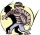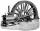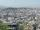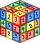# Curiosity factor

A blogger starts a new website, initially the number of the traffic is 293 due to their curiosity factor. The business owner estimated that the traffic will increase by 2,6% per week. What will be the number of it in week 5?.

Result

t =  333

#### Solution:Leave us a comment of example and its solution (i.e. if it is still somewhat unclear...):Be the first to comment!## Next similar examples:

1. Theorem proveWe want to prove the sentence: If the natural number n is divisible by six, then n is divisible by three. From what assumption we started?
2. The crimeThe crime rate of a certain city is increasing by exactly 7% each year. If there were 600 crimes in the year 1990 and the crime rate remains constant each year, determine the approximate number of crimes in the year 2025.
3. PowerNumber ?. Find the value of x.
4. A perineumA perineum string is 10% shorter than its original string. The first string is 24, what is the 9th string or term?
5. Geometric sequence 4It is given geometric sequence a3 = 7 and a12 = 3. Calculate s23 (= sum of the first 23 members of the sequence).
6. Five membersWrite first 5 members geometric sequence and determine whether it is increasing or decreasing: a1 = 3 q = -2
7. GP - 8 itemsDetermine the first eight members of a geometric progression if a9=512, q=2
8. Geometric progression 2There is geometric sequence with a1=5.7 and quotient q=-2.5. Calculate a17.
9. Six termsFind the first six terms of the sequence a1 = -3, an = 2 * an-1
10. Tenth memberCalculate the tenth member of geometric sequence when given: a1=1/2 and q=2
11. Profit gainIf 5% more is gained by selling an article for Rs. 350 than by selling it for Rs. 340, the cost of the article is:
12. MachinePrice of the new machine is € 62000. Every year is depreciated 15% of residual value. What will be the value of the machine after 3 years?
13. The city 2Today lives 167000 citizens in city. How many citizens can we expect in 11 years if their annual increase is 1%?
14. The cityAt the end of 2010 the city had 248000 residents. The population increased by 2.5% each year. What is the population at the end of 2013?
15. QuotientDetermine the quotient and the second member of the geometric progression where a3=10, a1+a2=-1,6 a1-a2=2,4.
16. EquationHow many real roots has equation ? ?
17. Simple interest 3Find the simple interest if 11928 USD at 2% for 10 weeks.